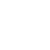Oregonator:
Filter:
Classes (extension) |

# Oregonator : MultiOutUGen : UGen : AbstractFunction : ObjectExtension

Chemical reaction modelling Oscillator
Source: SLUGens.sc

//SLUGens released under the GNU GPL as extensions for SuperCollider 3, by Nick Collins, http://composerprogrammer.com/index.html

## Description

Euler ODE solver implementation of the Oregonator equations (http://www.scholarpedia.org/article/Oregonator).

x' = epsilon* (q*y - x*y + x*(1-x) ) y' = mu* (-q*y -x*y +z) z' = x-y

All inputs can have .kr rate UGens plugged in.

The Oregonator can blow up, treat with caution.

## Class Methods

### Oregonator.ar(reset: 0, rate: 0.01, epsilon: 1.0, mu: 1.0, q: 1.0, initx: 0.5, inity: 0.5, initz: 0.5, mul: 1.0, add: 0.0)

#### Arguments:

 reset If > 0.0, restart with new initial conditions sampled from initx, inity, initz rate update rate for a sample step epsilon equation constant mu equation constant q equation constant initx reset value for x inity reset value for y initz reset value for z